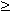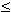### k-facet graphs revisited

Let k2 be a fixed integer. Random simple k-uniform hypergraphs are easy and natural to define, just choose some function p(n) with 0 < p(n) < 1 , and let Hk(n,p) be the random hypergraph with vertex set {1,2,...,n} where each k-element subsets has independently the same chance p(n) to occur as hyperedge.

We shall show that for suitable p(n) = n^{-1/2+},>0, k-facet graphs of Hk(n,p) are almost always recognizable, for n going to infinity. Each of both remaining problems---identifying the star graphs and recognizing (k-1)-set hypergraphs of k-uniform hypergraphs---becomes tractable almost always.

For the first we could show that for almost every such k-facet graph, all its star cliques have more than k+1 vertices.

For the other part, testing whether a given simple, linear k-uniform hypergraph is the (k-1)-set hypergraph of some simple k-uniform hypergraph H, we try the following approach: Assume H´ = H[k-1]. Under this assumption, in the first step we try to find out which pairs of vertices of have---viewed as k-1-element subsets of A(H)---k-2 common elements. These pairs are sampled as edges of the graph F=(A(H´),E). If this has been done, we only need to check in the second step whether the resulting graph is a (k-2)-intersection graph of some (k-1)-uniform hypergraph. This second step sounds difficult enough, but for k=3 we can do it; it is just recognizing line graphs.

If two distinct (k-1)-element subsets are both contained in the same k-element superset, then we have exactly k-2 common elements. Thus

(1) all pairs of vertices lying in the same hyperedge of are included in E.

However, we may have to include more.

Next assume that the vertices x and y of are in no common hyperedge, but that another vertex z joins a common hyperedge with x and another common hyperedge with y. If X,Y, and Z are the corresponding (k-1)-element subsets of A(H), then |XZ|=k-2 and |YZ| = k-2, thus |XY|k-3. If |XY|=k-3, at most four such vertices z are possible. Thus, if there are at least five such vertices z, then we know |XY|=k-2 and add xy to our edge set E.

(2) If there are distinct vertices x,y,z1,z2,z3,z4, z5 in where for every 1i5 some hyperedge of contains both x and zi, and another hyperedge contains both y and zi, then we add xy to E.

There may be, however, still more edges in E.

Not so if H is random, H=Hk(n,p), with large enough n and high enough edge probability p.Let H´ =Hk(n,p)[k-1], where p=p(n)= n^{-1/2+}, for>0. Then, almost surely, the graph F constructed by the two rules (1) and (2) above, is connected, and F is the (k-2)-intersection graph of the (k-1)-uniform hypergraph H´´ whose hyperedges are all (k-1)-element subsets of the hyperedges of Hk(n,p).

For recognizing 3-facet graphs of large random 3-uniform hypergraphs we simply decide that all cliques with more than 4 vertices are star graphs. We test whether this hypergraph is the 2-set hypergraph of some 3-uniform hypergraph, and are done. The only problem is that the hypergraph of the star graphs is not really random, but it can be shown that it behaves random. For testing k-facet graphs with k4, we do the same and arrive at the problem of recognizing (k-1)-facet graphs of Hk-1(n,1), which works quite well.

Erich Prisner